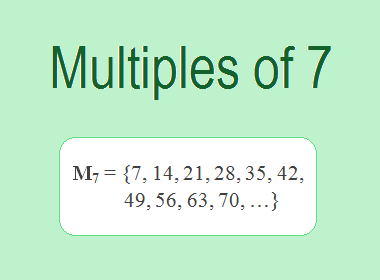# Multiples of 7The Multiples of 7 are 7, 14, 21, 28, 35, 42, 49, 56, 63, 70, … i.e. M7 = {7, 14, 21, 28, 35, 42, 49, 56, 63, 70, …}. Multiples of 7 are the series of numbers obtained by multiplying 7 with the natural numbers.

7 × 1 = 7

7 × 2 = 14

7 × 3 = 21

7 × 4 = 28

7 × 5 = 35

7 × 6 = 42

7 × 7 = 49

7 × 8 = 56

7 × 9 = 63

7 × 10 = 70

And so on.

From this series, we observe that the multiples of 7 can be derived by multiplying 7 by natural numbers in ascending order. These multiples form an arithmetic sequence with a common difference of 7.

## Properties of Multiples of 7

Multiples of 7 possess several interesting properties that make them unique. Here are a few notable ones:

1.    Divisibility by 7: Every multiple of 7 is divisible by 7 without leaving a remainder. This property holds true for all multiples of 7 since they are formed by multiplying 7 by an integer.

2.    Last Digit Cycles: A fascinating property of multiples of 7 is their cyclic behavior when it comes to the last digit. The last digit of each multiple follows a predictable pattern that cycles through 7, 4, 1, 8, 5, 2, and 9. For example, the last digit of 7 is 7, while the last digit of 14 is 4, and so on. This cyclic pattern repeats indefinitely as we generate more multiples of 7.

3.    Increasing by 7: Similar to other multiples, each subsequent multiple of 7 is obtained by adding 7 to the previous multiple. Starting from 7, we add 7 to obtain 14, then 21, and so on. This pattern of adding 7 continues infinitely, generating a sequence of multiples that increases steadily.

********************

********************

## Patterns and Observations:

1.    Three-Digit Cycles: When examining multiples of 7, we notice that the last three digits of consecutive multiples form a repeating pattern. For example, the last three digits of 7 are 007, while the last three digits of 14 are 014. This three-digit cycle repeats indefinitely, allowing us to anticipate the last three digits of any multiple of 7.

2.    Alternating Parity: Another intriguing pattern emerges when considering the parity of multiples of 7. The parity alternates between odd and even numbers. For instance, 7 itself is an odd number, while 14 is even, followed by 21 (odd), 28 (even), and so on. This alternating pattern of odd and even multiples continues infinitely.

## Applications of Multiples of 7

The study of multiples of 7 finds applications in various fields, including mathematics, science, and everyday life:

1.    LCM: One of the applications of multiples of 7 is in finding the lowest common multiple (LCM) of two or more numbers. The LCM is the lowest multiple that two or more numbers have in common. For example, to find the LCM of 7 and 5, we need to find the multiples of both numbers and identify the lowest multiple they have in common. The multiples of 7 are 7, 14, 21, 28, 35, 42, 49, 56, 63, 70, etc. The multiples of 5 are 5, 10, 15, 20, 25, 30, 35, 40, 45, 50, … etc. The lowest multiple that they have in common is 35. Therefore, the LCM of 7 and 5 is 12.

2.    Calendar Systems: Multiples of 7 play a crucial role in constructing calendar systems. For instance, the Gregorian calendar, widely used today, has a 7-day week, with multiples of 7 forming the basis for organizing time.

3.    Divisibility Tests: The knowledge of multiples of 7 can be employed as a divisibility test. If a number is a multiple of 7, it is divisible by 7. This fact can be useful in simplifying calculations and identifying divisibility patterns.

4.    Mathematical Puzzles and Games: Multiples of 7 often appear in mathematical puzzles, riddles, and games. They serve as key elements in generating sequences, determining patterns, and providing challenges for problem-solving enthusiasts.

## Conclusion:

The Multiples of 7 are the numbers obtained by multiplying 7 with natural numbers. The multiples of 7 are 7, 14, 21, 28, 35, 42, 49, 56, 63, 70, etc. The multiples of 7 have various properties, such as divisibility by 7, last digit cycles, increasing by 7, three-digit cycles, alternating parity, etc. The multiples of 7 have several applications in mathematics, such as finding the LCM, calendar systems, divisibility tests, and mathematical puzzles and games.